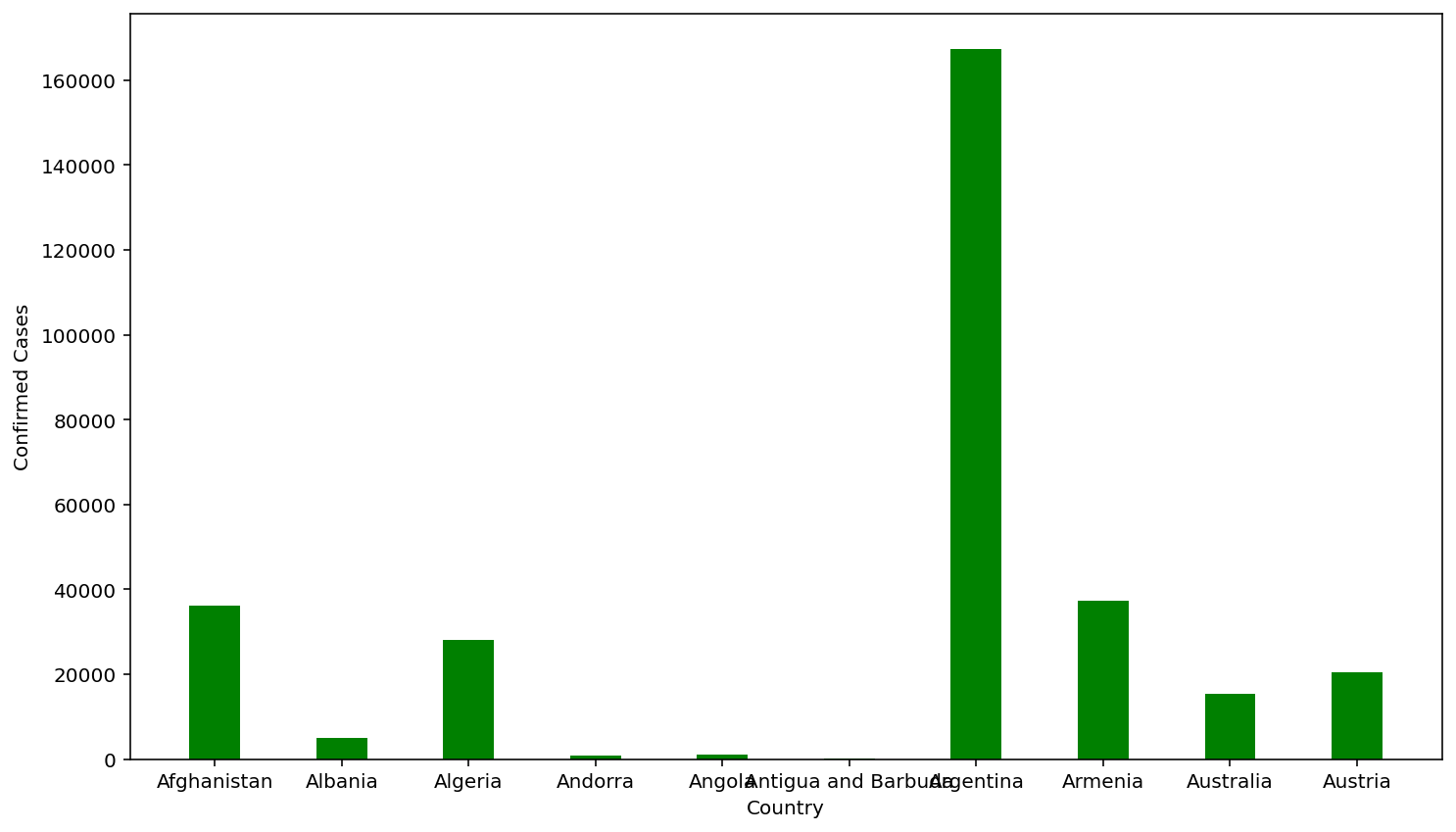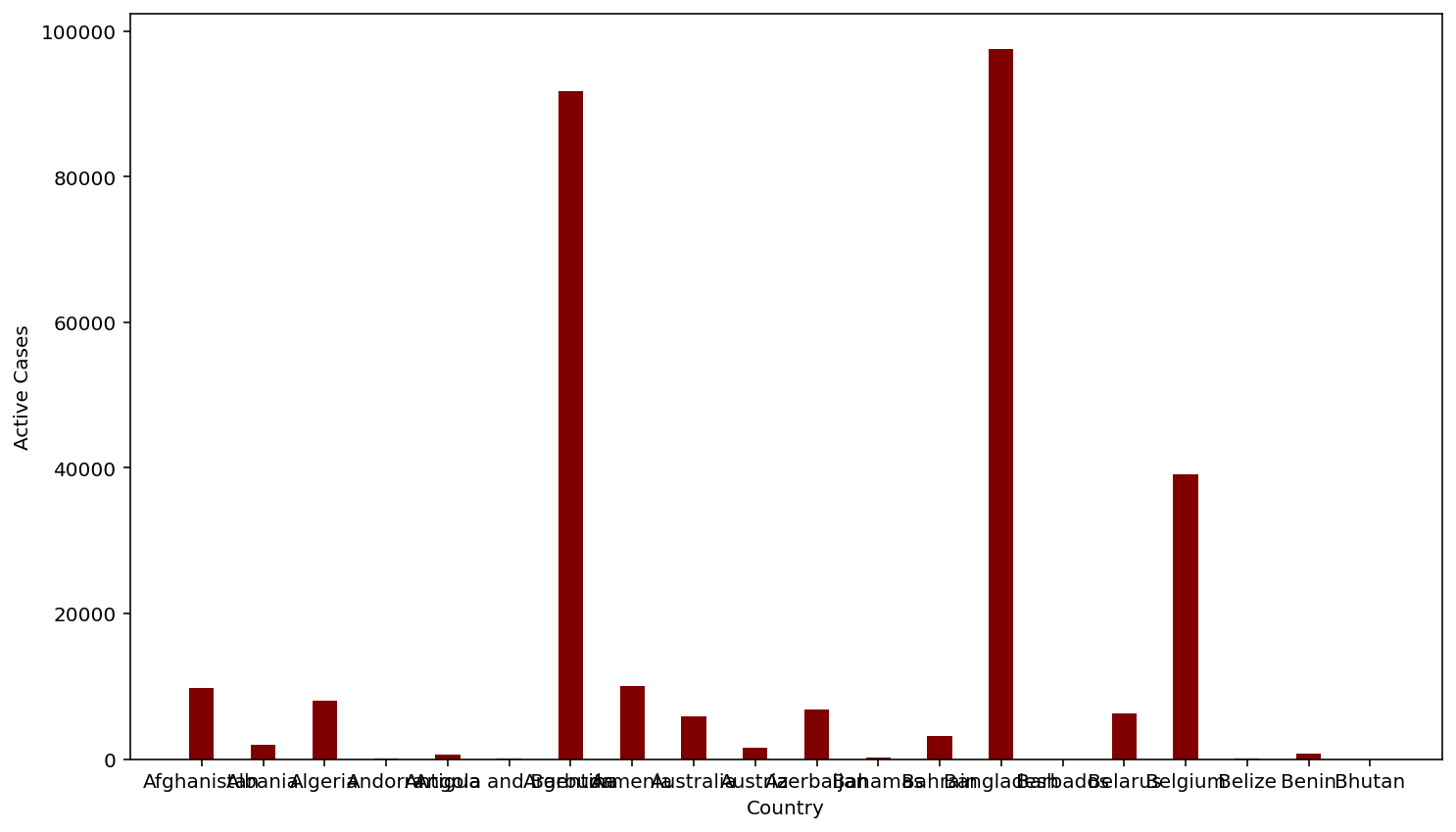Open In App

# How to Change the Color of a Graph Plot in Matplotlib with Python?

Prerequisite: Matplotlib

Python offers a wide range of libraries for plotting graphs and Matplotlib is one of them. Matplotlib is simple and easy to use a library that is used to create quality graphs. The pyplot library of matplotlib comprises commands and methods that makes matplotlib work like matlab. The pyplot module is used to set the graph labels, type of chart and the color of the chart. The following methods are used for the creation of graph and corresponding color change of the graph.

Syntax: matplotlib.pyplot.bar(x, height, width, bottom, align, **kwargs)

Parameter:

• x : sequence of scalers along the x axis
• height : sequence of scaler determining the height of bar ie y-axis
• width : width of each bar
• bottom : Used to specify the starting value along the Y axis.(Optional)
• align : alignment of the bar
• **kwargs : other parameters one of which is color which obviously specifies the color of the graph.

Return Value: Returns the graph plotted from the specified columns of the dataset.

Link to the dataset: Corona virus report

Example 1:

## Python3

 `# import packages``import` `pandas as pd``import` `matplotlib``import` `matplotlib.pyplot as plt`` ` `# import dataset``df ``=` `pd.read_csv(``'country_wise_latest.csv'``)`` ` `# select required columns``country ``=` `df[``'Country/Region'``].head(``10``)``confirmed ``=` `df[``'Confirmed'``].head(``10``)`` ` `# plotting graph``plt.xlabel(``'Country'``)``plt.ylabel(``'Confirmed Cases'``)``plt.bar(country, confirmed, color``=``'green'``, width``=``0.4``)`` ` `# display plot``plt.show()`

OutputExample 2:

## Python3

 `# import packages``import` `pandas as pd``import` `matplotlib``import` `matplotlib.pyplot as plt`` ` `# import dataset``df ``=` `pd.read_csv(``'country_wise_latest.csv'``)`` ` `# select required data``country ``=` `df[``'Country/Region'``].head(``20``)``confirmed ``=` `df[``'Active'``].head(``20``)`` ` `# plot graph``plt.xlabel(``'Country'``)``plt.ylabel(``'Active Cases'``)``plt.bar(country, confirmed, color``=``'maroon'``, width``=``0.4``)`` ` `# display plot``plt.show()`

Output